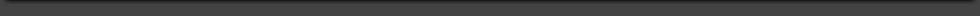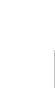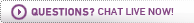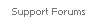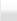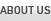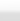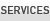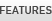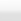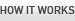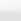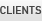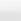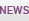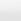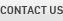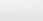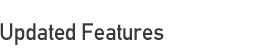Multiple Looping Filters in a Function Question

Similar to the multiple looping validations, we now allow you to eliminate the repetition associated with duplicate filters in an Equation Multiple Value function question. You can use this feature any time you create function question filters than only change the row number.

For example, in a row question, if you want to captures all rows that are greater than 3 and pull them into a follow up question, create the following equation multiple value function question with one filter: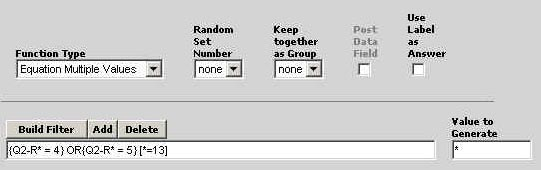This example assumes we are working on question 2 with 13 rows, and we want to capture all rows with an answer greater than 3.

Multiple Looping Filter Syntax

 Syntax Explanation * Replaces the row number [*=13] Indicates number of rows * Creates the corresponding number in the value to generateSurveyWriter.Com © 2015 | Privacy Policy | Terms Of Use